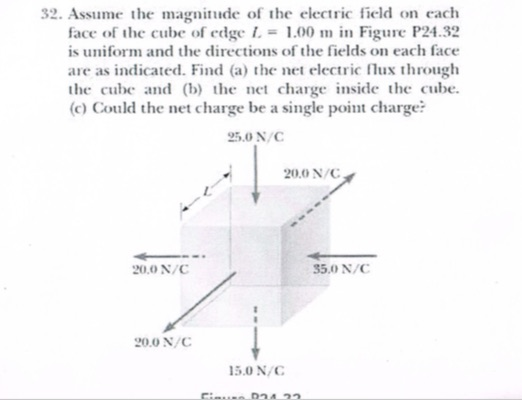# 32. Assume the magnitude of the electric field on eachface of the cube of edge1.00 m in Figure P24.32is uniform and the directions of the fields on each faceare as indicated. Find (a) the net electric lux throughthe cube and ( the net charge inside the cube.(c) Could the net charge be a single point charge?ON/C200 N/C200 N/C850N/C200N/C5.ON/C

Questionhelp_outlineImage Transcriptionclose32. Assume the magnitude of the electric field on each face of the cube of edge1.00 m in Figure P24.32 is uniform and the directions of the fields on each face are as indicated. Find (a) the net electric lux through the cube and ( the net charge inside the cube. (c) Could the net charge be a single point charge? ON/C 200 N/C 200 N/C 850N/C 200N/C 5.ON/C fullscreen
check_circleExpert Solution
Step 1

a)

First, we determine the area of each face of the cube. length of each side of the cube is given as 1 m.  Hence the area of each face of the cube is given as

a = Length of side of the cube = 1 m

A = Area of each face of the cube

Hence the area of each face of the cube comes out to be 1 m2 and the direction of the area at any face is normal to the surface and going away from it.

Step 2

For left and right face :

Assuming left-right direction along the x-axis with the left being negative x-direction and right being the positive x-direction.

Step 3

For top and bottom face :

Assuming top-bottom direction along the z-axis with the down b...

### Want to see the full answer?

See Solution

#### Want to see this answer and more?

Solutions are written by subject experts who are available 24/7. Questions are typically answered within 1 hour*

See Solution
*Response times may vary by subject and question
Tagged in

### Physics